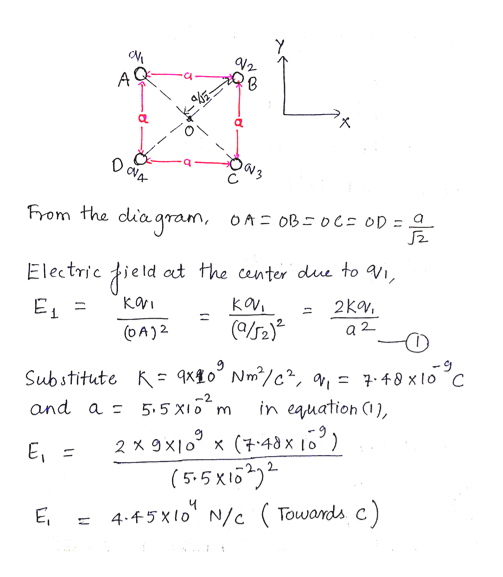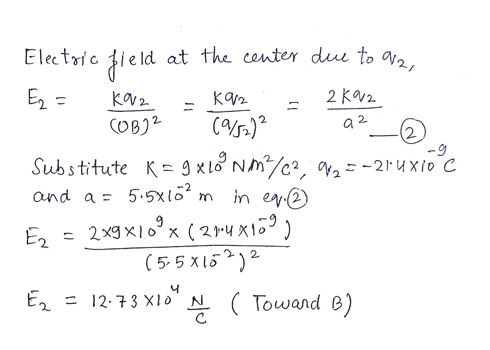42a

Question

In the figure the four particles form a square of edge length a = 5.50 cm and have charges q1 = 7.48 nC, q2 = -21.4 nC, q3 = 21.4 nC, and q4 = -7.48 nC. What is the magnitude of the net electric field produced by the particles at the square's center?

Step 1

Given:

Charge q1 = 7.8 nC

Charge q2 = -21.4 nC

Charge q3 = 21.4 nC

Charge q4 = - 7.8 nC

Length of the side of the square, a = 5.5 cm

Step 2

Calculating the electric field at the center O due to charge q1:help_outlineImage TranscriptioncloseA From the dia gram, OA OB oCs OD Electric ield at the center due to V1, KOV (0s2) KoVi 2Kv EL a 2 (OA) 2 -9 Substitute K = qx£o° Nm°/c^, ^, in eauation (), -2 and a 5.5 X10 m 2 x 9Xlo x (4d x 1o) (5-5 xi62 E, 4.45xlo N/c Towards c) 1 fullscreen
Step 3

Calculating the electric field at th...help_outlineImage Transcriptioncloseat the center du to 2 Electic E = 2 Kv2 Kav2 KV2 CUB2 a 2 -9 Substitute K g x lNc in en -2xio C -2 and a 5 5x1 2xg Xlox (21-ų xio ( 5-5X15 E2 E2 12-73 X1o" N Towand e) fullscreen

Want to see the full answer?

See Solution

Want to see this answer and more?

Our solutions are written by experts, many with advanced degrees, and available 24/7

See Solution
Tagged in

Physics